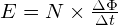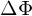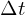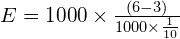# Faraday’s law of electromagnetic induction | Easiest Explanation

Faraday’s law of electromagnetic induction | Easiest Explanation 1024 576 Gaurav J

While experimenting, Michael faraday made a very important discovery in electromagnetism. Now, known as faraday’s law of electromagnetic induction. Faraday’s discovery has given birth to application like Transformer, Generators, AC motors. Therefore, it is very important to every electrical engineer to understand this law, as it will make learning of transformers, generators and AC motor very easy. So, let’s start.

Michael faraday, revealed a fundamental relationship between the voltage and flux in the circuit. So, let’s see what exactly he revealed. He discovered two important things.

#1: If the flux linking a loop or turn, varies as a function of time, a voltage is induced between its terminal.

This means that, let’s say you have a copper coil. Which is surrounded with flux, and if you vary the flux, a voltage will get induced across the terminal of that coil.

Now, it really doesn’t matter if you vary the flux or coil.
But, it is also important to know what amount of voltage will get induced? And on what basis the value of induced voltage depends? We don’t have to worry on that, as faraday has already discovered that. And which is the 2nd statement of faraday’s law of electromagnetic induction.

#2: The value of induced voltage is proportion to the rate of change of flux.

The faster the flux will vary the more voltage will generate and vice versa.
We can put this statement in a mathematical form also. If flux varies inside of a coil of N turn, the voltage induced is given by,Where,
E = Induced voltage (v)
N = Number of turns= Change in the flux inside the coil= time interval during which the flux changes

Let’s take an example. You have a coil with 1000 turns which is surrounded by flux of 6 mWb produced by a permanent magnet as shown in fig A.

Now, the magnet is suddenly moved (fig.B) and the flux inside the coil reduced to 3 mWb in 1/10 of a second. What will be the voltage induced?We can use the above formula to find out the total amount of voltage induced. Let’s put the values in the formula.E = 30 volts

Faraday’s law of electromagnetic induction has given birth to many application like transformer, generator and AC motors. This law is the basis of the working of these equipment, and that’s why this law is very very important. Now, as you have understood this law, I am sure that you will also understand the working principal of transformer, generators and AC motors.###### Gaurav J

Electrical Engineer. Content Creator. Currently working with a High & Extra High Voltage Switchgear Industry.

All stories by: Gaurav J